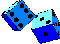## Conclusion

This workbook offers several simulation models that are appropriate for use of three common research designs for evaluating program effects. The logic of these simulations can be easily extended to other relevant research contexts. For instance, many agencies routinely conduct sample surveys to identify needs and target populations, assess services that are provided, and compare agency functioning with the performance of other similar agencies or with some standard. One would construct simulation models for survey instruments for the same reasons that they are constructed for evaluation designs--to improve teaching and general understanding, to explore problems in implementing the survey (such as non response patterns), or to examine the probable effect of various analytic strategies. The key to doing this would again rest on the statistical model used to generate hypothetical survey responses. A "true score" measurement model is useful, at least for simple simulations, but may have to be modified. For instance, assume that one question on a survey deals with client satisfaction with a particular service and that the response is a 7-point Likert-type format where 1=very dissatisfied, 7=very satisfied, and 4=neutral. The analyst could make the assumption that for some sample or subsample the true average response is a scale value equal to 5 points (somewhat satisfied), and that the true distribution of responses is normal around these values, with some standard deviation. At some point, the analyst will have to convert this hypothetical underlying continuous true distribution to the 7-point integer response format either by rounding or by generating normally distributed random integers in the first place. Such a variable could then be correlated or cross-tabulated with other generated responses to explore analytic strategies for that survey. Similar extensions of the models discussed here can be made for simulations of routinely collected management information system (MIS) information, for data for correlational studies, or for time-series situations, among others.

Simulations are assumptive in nature and vary in quality to the degree that the reality is correctly modeled. When constructing a simulation, it is important that the analyst seek out empirical evidence to support the assumptions that are made whenever this is feasible. For instance, it should be clear that the simulations described here could be greatly enhanced if we had more specific data on how much and what type of attrition typically occurs, what type of floor or ceiling effects are common, what patterns of misassignment relative to the cutoff value typically arise for the RD design, what the typical test-retest reliabilities (for use in reliability-corrected ANCOVA) might be, and so on. Although some relevant data will be available in the methodological literature, all of these issues are context specific and demand that the analyst know the setting in some detail if the simulations are to be reasonable.

One way to approach the assumptive nature of the simulation task is to recognize that reality conditions or constraints in the models need to be examined systematically across a range of plausible conditions. This implies that multiple analyses under systematically varied conditions that are based upon principles of parametric experimental design are needed in state-of-the art simulation work. This point is made well by Heiberger et al. (1983:585):

The computer has become a source of experimental data for modern statisticians much as the farm field was to the developers of experimental design. However, many "field" experiments have largely ignored fundamental principles of experimental design by failing to identify factors clearly and to control them independently. When some aspects of test problems were varied, others usually changed as well--often in unpredictable ways. Other computer-based experiments have been ad hoc collection of anecdotal results at sample points selected with little or no design.

Heiberger et al. (1983) go on to describe a general model for simulation design that allows the analyst to control systematically a large number of relevant parameters across some multidimensional reality space, including the sample size, number of endogenous variables, number of "key points" or condition values, matrix eigenvalues and eigenvectors, intercorrelations, least squares regression coefficients, means standard errors, and so on.

Although rigorous, experimentally based simulations are essential for definitive analysis of complex problems, they will not always be feasible or even desirable for many program evaluation contexts. Instead, it is important to recognize that simulations are generally a useful tool that can be used to conduct more definitive statistical studies. However, simulations in program evaluation can provide the analyst with the means to explore and probe simple relevant data structures for the purposes of improving the instruction of research, examining research implementation issues and pilot testing analytic approaches for problematic data.Simulation Home Page